﻿ Varipend®contents:

Engl

Find: in
About an opportunity of "reactionless" moving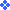2.  Article2.2.  The analysis of movement of the closed mechanical system, using a principle of conservation of momentum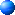2.2.1. Calculation of a linear momentum of system of mobile elements in relative coordinates system.

Linear momentum of material system to equally mass of all system increased for speed of its center of inertia. In our case: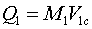(2)

Here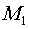Is a mass of all elements of the system moving on a circle. From conditions of a task follows, that during the initial moment of time the mass is distributed in regular intervals. From here it is possible to accept: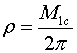(3)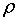Angular density of distribution of elements of system of bodies on a circle (an arch of a circle).

Sizechanges linearly in time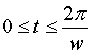: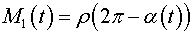(4)

whence: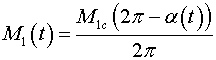(5)

or, as dependence on a corner of filling:It is possible to write down also: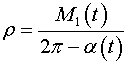Size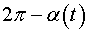defines a corner on a trajectory filled by moving elements.

In case of our task, the center of mass of system of mobile elements in coordinates system XOY it is calculated:,

where xi , yi -coordinates i-th site of a body in mass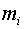.

Coordinates i-th mobile element in the chosen coordinates system: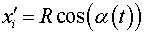From conditions of our task: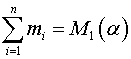Coordinates of the center of mass of mobile elements in the chosen coordinates system are calculated by means of integrals with a variable bottom limit:(6)

After integration we shall receive:(7)(8)

Expressions (7) and (8) define coordinates of the center of mass of mobile elements depending on a corner of an aperture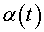. From a condition of a task the corner of an aperture changes linearly eventually:The plot of moving center of mass of system of mobile elements is presented in a Fig. 2:Fig. 2

In a Fig. 3 it is shown conditional  movings of the center of mass of mobile system.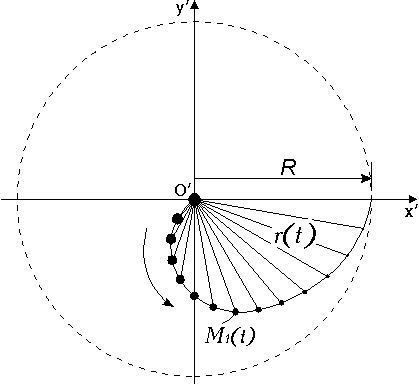Fig. 3:

Moving of the center of mass of system of mobile elements to coordinates system XOY it is possible to compare to moving the center of mass of a pendulum of variable lengthand variable mass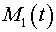.

In an animated kind it looks as follows:

In a projection to axes of coordinates system  XOY a linear momentum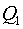: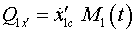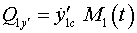(9)(10)

In a projection to axes of coordinates system XOY a linear momentum:(11)(12)

, where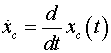projections of speed of the beginning of coordinates of system XOY on corresponding axes of system XOY.

Let's remind: the beginning of coordinates of system XOY it is connected with the center of mass of a body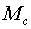////
Сайт создан в системе uCoz